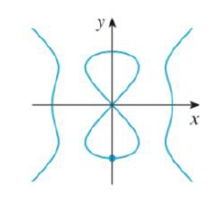Chapter 3.5, Problem 32E### Single Variable Calculus: Early Tr...

8th Edition
James Stewart
ISBN: 9781305270343

#### Solutions

Chapter
Section### Single Variable Calculus: Early Tr...

8th Edition
James Stewart
ISBN: 9781305270343
Textbook Problem

# Use implicit differentiation to find an equation of the tangent line to the curve at the given point.32. y2(y2 – 4) = x2(x2 –5), (0. –2), (devil’s curve)To determine

To find: The equation of the tangent line to the given equation at the point.

Explanation

Given:

The curve is y2(y24)=x2(x25).

The point is (0,2).

Derivative rules:

(1) Chain rule: If y=f(u) and u=g(x)  are both differentiable function, then dydx=dydududx.

(2) Product rule: ddx(f(x)g(x))=f(x)ddx(g(x))+g(x)ddx(f(x))

Formula used:

The equation of the tangent line at (x1,y1) is, yy1=m(xx1) (1)

Where, m is the slope of the tangent line at (x1,y1) and m=dydx|x=x1,y=y1.

Calculation:

Obtain the equation of the tangent line to the equation at the point.

Rewrite the given equation as follows,

y2(y24)=x2(x25)y44y2=x45x2

Differentiate the equation implicitly with respect to x,

ddx(y44y2)=ddx(x45x2)ddx(y4)4ddx(y2)=ddx(x4)5ddx(x2)ddx(y4)4ddx(y2)=4x210x<

### Still sussing out bartleby?

Check out a sample textbook solution.

See a sample solution

#### The Solution to Your Study Problems

Bartleby provides explanations to thousands of textbook problems written by our experts, many with advanced degrees!

Get Started

#### Simplify the expressions in Exercises 97106. x1/2yx2y3/2

Finite Mathematics and Applied Calculus (MindTap Course List)

#### In Exercises 4562, find the values of x that satisfy the inequality (inequalities). 59. x+3x20

Applied Calculus for the Managerial, Life, and Social Sciences: A Brief Approach

#### In problems 45-62, perform the indicated operations and simplify. 62.

Mathematical Applications for the Management, Life, and Social Sciences

#### Find the derivative of the function. g(x)=ex2x

Single Variable Calculus: Early Transcendentals

#### Factor: 7xy21y

Elementary Technical Mathematics